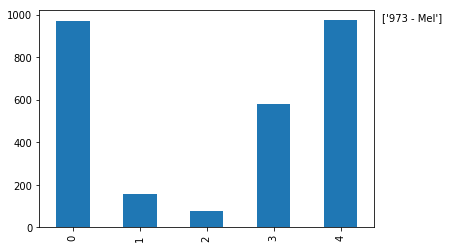# 学习Pandas，第 1 课

``````# 导入所有需要的库

# 导入一个库中制定函数的一般做法:
##from (library) import (specific library function)

# 导入一个库的一般做法:
##import (library) as (give the library a nickname/alias)
import matplotlib.pyplot as plt
import pandas as pd #导入pandas的常规做法
import sys #导入sys库只是为了确认一下Python的版本
import matplotlib #这样导入matplotlib只是为了显示一下其版本号

# 初始化matplotlib，用inline方式显示图形
%matplotlib inline
``````
``````print('Python version ' + sys.version)
print('Pandas version ' + pd.__version__)
print('Matplotlib version ' + matplotlib.__version__)
``````
``````Python version 3.6.1 | packaged by conda-forge | (default, Mar 23 2017, 21:57:00)
[GCC 4.2.1 Compatible Apple LLVM 6.1.0 (clang-602.0.53)]
Pandas version 0.19.2
Matplotlib version 2.0.2
``````

## 创建数据

``````# 初始数据集: 婴儿名字和出生率
names = ['Bob','Jessica','Mary','John','Mel']
births = [968, 155, 77, 578, 973]
``````

zip 函数将这两个列表合并在一起。

``````# 查看一下zip函数的帮助
zip?
``````
``````BabyDataSet = list(zip(names, births))
BabyDataSet
``````
``````[('Bob', 968), ('Jessica', 155), ('Mary', 77), ('John', 578), ('Mel', 973)]
``````

df 是一个 DataFrame对象。 你可以把这个对象理解为包含了 `BabyDataset` 的内容而格式非常象一个 sql 表格或者 Excel 的数据表。 让我们看看 df 中的内容。

``````df = pd.DataFrame(data = BabyDataSet, columns=['Names', 'Births'])
df
``````
Names Births
0 Bob 968
1 Jessica 155
2 Mary 77
3 John 578
4 Mel 973

``````# 查看一下 to_csv 的帮助
df.to_csv?
``````

``````df.to_csv('births1880.csv', index=False, header=False)
``````

## 获取数据

``````read_csv?
``````

``````Location = r'./births1880.csv' #从 notebook 当前的位置读取 csv 文件
``````

``````df
``````
Bob 968
0 Jessica 155
1 Mary 77
2 John 578
3 Mel 973

``````df = pd.read_csv(Location, header=None)
df
``````
0 1
0 Bob 968
1 Jessica 155
2 Mary 77
3 John 578
4 Mel 973

``````df = pd.read_csv(Location, names=['Names', 'Births'])
df
``````
Names Births
0 Bob 968
1 Jessica 155
2 Mary 77
3 John 578
4 Mel 973

``````import os
os.remove(Location)
``````

## 准备数据

Names 列是由字母和数字串组成的婴儿名字。 这一列也许会出现一些脏数据但我们现在不需要有太多顾虑。 Births 列应该是通过整型数字(integers)表示一个指定年份指定婴儿名字的出生率。 我们可以检查一下是否这一列的数字都是整型。这一列出现浮点型(float)是没有意义的。但我们不需要担心这一列出现任何可能的离群值(outlier)。

``````# 查看每一列的数据类型
df.dtypes
``````
``````Names     object
Births     int64
dtype: object
``````
``````# 查看 Births 列的数据类型
df.Births.dtype
``````
``````dtype('int64')
``````

## 分析数据

• 将 dataframe 排序并且找到第一行
• 使用 max() 属性找到最大值
``````# 方法 1:
Sorted = df.sort_values(['Births'], ascending=False)
``````
Names Births
4 Mel 973
``````# 方法 2:
df['Births'].max()
``````
``````973
``````

## 表现数据

pandas 使用非常方便的 plot() 让你用 dataframe 中的数据来轻松制图。 刚才我们在 Births 列找到了最大值，现在我们要找到 973 这个值对应的婴儿名字。

df['Names'] - 这是完整的婴儿名字的列表，完整的 Names 列
df['Births'] - 这是1880年的出生率，完整的 Births 列
df['Births'].max() - 这是 Births 列中的最大值

`[df['Births'] == df['Births'].max()]` 的意思是 [在 Births 列中找到值是 973 的所有记录]
`df['Names'][df['Births'] == df['Births'].max()]` 的意思是 在 Names 列中挑选出 [Births 列的值等于 973] (Select all of the records in the Names column WHERE [The Births column is equal to 973])

str() 可以将一个对象转换为字符串。

``````# 绘图
# df['Births'].plot()
df['Births'].plot.bar()  #这里改用的条形图更直观

# Births 中的最大值
MaxValue = df['Births'].max()

# 找到对应的 Names 值
MaxName = df['Names'][df['Births'] == df['Births'].max()].values

# 准备要显示的文本
Text = str(MaxValue) + " - " + MaxName

# 将文本显示在图形中
plt.annotate(Text, xy=(1, MaxValue), xytext=(8, 0),
xycoords=('axes fraction', 'data'), textcoords='offset points')

print("The most popular name")
df[df['Births'] == df['Births'].max()]
``````
``````The most popular name
``````
Names Births
4 Mel 973This tutorial was created by HEDARO

``````